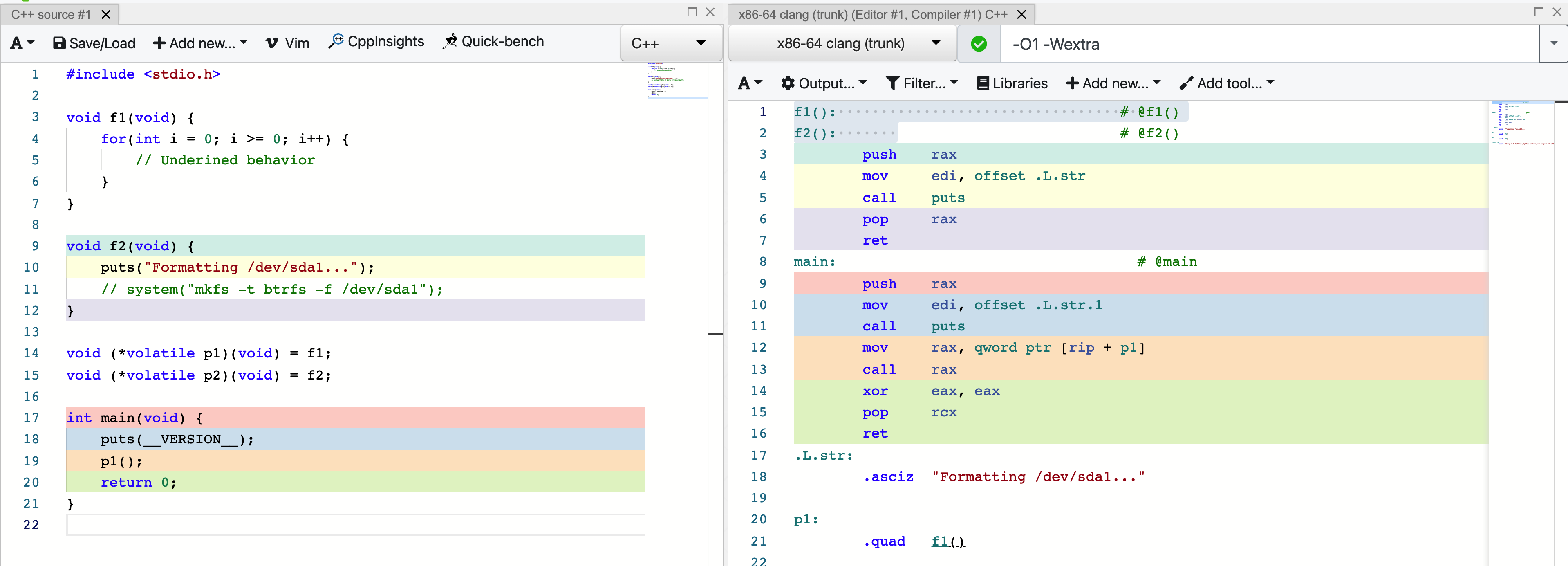Leo's cottage.

0%

## 背景

1. 这段代码运行结果和本地不一样
while (head->next != nullptr) {
if (cnt == m) {
cout << p->data << ' ';

// del 是代码作者定义的一个函数，里面调用了
// delete p
del(p);
cnt = 0;
} else {
cnt += 1;
if (p->next != nullptr) {
p = p->next;
} else {
}
}
}


1. 这段更离谱：
template<typename T> bool ArrayList<T>::append(T const& value) {
if(_size >= _capacity){ // border check;
cout<<"SIZE "<<_size<<" CAP "<<_capacity;
cout << " The List is overflow!" << endl;
return false;
}
_elem[_size++] = value;
}

1. 这段代码很难找出错（所以建议跳过去不看）：
#include <iostream>
using namespace std;
int main() {
int n, s, m, j, tempt, q, k, flag;
int count = 1;
int data;
cin >> n >> s >> m;
tempt = s;
for (int i = 0; i < n; i++) {
data[i] = i + 1;
}
for (; count <= n; count++) { // 主要看这个for循环
for (k = 1, q = 0; k <= m; q++) {
if (data[(tempt + q - 1) % n] != 0)
k++;
}
if (count != n) {
cout << data[(tempt + q - 2) % n];
cout << ' ';
} else {
cout << data[(tempt + q - 2) % n];
return 0;
}
data[(tempt + q - 2) % n] = 0;
for (j = 1, flag = 0; flag != 1; j++) {
if (data[(tempt + q + j - 2) % n] != 0)
flag = 1;
else
flag = 0;
}
tempt = (tempt + q + j - 2) % n;
}
return 0;
}

$clang++ a.cpp -Wall --std=c++17 -fsanitize=undefined$ ./a.out
6 1 1 // 这行是输入的
a.cpp:14:9: runtime error: index -1 out of bounds for type 'int '
SUMMARY: UndefinedBehaviorSanitizer: undefined-behavior a.cpp:14:9 in
1 2 3 4 5 6

## 什么是未定义行为

1. 数组越界访问
2. 解引用空指针
3. 在对象的生命周期结束后访问对象
4. 有返回类型的函数没有从return结束

• 未定义行为（undefined behavior，UB）——对程序的行为无任何限制。未定义行为的例子是数组边界外的内存访问，有符号整数溢出，空指针的解引用，在一个表达式中对同一标量多于一次的中间无序列点 (C++11 前)无序 (C++11 起)的修改，通过不同类型的指针访问对象，等等。编译器不需要诊断未定义行为（尽管许多简单情形确实会得到诊断），而且所编译的程序不需要做任何有意义的事。

template<typename T> bool ArrayList<T>::append(T const& value) {
if(_size >= _capacity){ // border check;
cout<<"SIZE "<<_size<<" CAP "<<_capacity;
cout << " The List is overflow!" << endl;
return false;
}
_elem[_size++] = value;
}

template<typename T> bool ArrayList<T>::append(T const& value) {
cout<<"SIZE "<<_size<<" CAP "<<_capacity;
cout << " The List is overflow!" << endl;
return false;
}

1. if内条件不成立的话，行为未定义。
2. 未定义行为不好，写代码的人会避免未定义行为。
3. 因此，写代码的人会保证每次调用的时候if内条件均成立。
4. 因此可以去掉if

• 如果if内条件成立，这样的执行结果自然是正确的
• 如果if内条件不成立，那么行为未定义。既然行为未定义，那任何行为都是正确的，因此我执行if内条件成立的代码也是正确的行为。

## 未定义行为的危害llvm的issue tracker中有关这个 “bug”的讨论还在进行中。一部分人认为应该“修复”：

This means UB is a potential safety/security problem, and we really should do something about it.

All sorts of UB manifests in lots of security issues, right? Buffer overruns and the like (I guess this is a buffer overrun, of sorts).

It’s not generally that simple - deciding where/how to “recover” from UB would be pretty difficult.

The Clang-advised way to deal with this would be to compile with -fsanitize=undefined

https://godbolt.org/z/3aW69c

example.cpp:4:29: runtime error: signed integer overflow: 2147483647 + 1 cannot be represented in type ‘int’
SUMMARY: UndefinedBehaviorSanitizer: undefined-behavior example.cpp:4:29 in